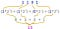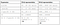How big is an int in Go?

Go has several built-in numeric types, “sets of integer or floating-point values.” Some architecture-independent types are uint8 (8-bit, unsigned integer), int16 (16-bit, signed integer), and complex128 (128-bit complex number). Curiously, the Go spec also includes architecture-dependent types:

• uint
• int
• uintptr

How many bits do these types require? Unhelpfully, the spec says int and uint are the same size, “either 32 or 64 bits.” A uintptr is “an unsigned integer large enough to store the uninterpreted bits of a pointer value.”

By far the most common of these three types I’ve seen is int. If you know your program will only use non-negative numbers, you could use uint instead. The most common time to use uintptr is when you’re importing unsafe, which is as safe to use as it sounds.

Practicalities

If these types are architecture-dependent, how can we tell how big an int is for an architecture we care about? One way is to go through the compiler source code, mapping your target architecture to its corresponding bit size. But, there are at least two solutions better than this:

• Use architecture-independent types. If you need to know exactly how many bits an int is using in your program, don’t rely on the whims of the compiler — use one of the predefined types (for example, int64)! This communicates your intent as the author for a variable to have a particular size (the size is in the type’s name!), improving readability and saving mental resources when your code is read later.
• Write a program to tell you. The standard library’s strconv package contains a helper to let you know how large an int is. strconv.IntSize “is the size in bits of an int or uint value.” You can use it in your program like this:

On 32-bit architectures, this will print 32. On 64-bit architectures, it will print 64. Check it out on the Playground, your computer, or in a 32-bit Docker image (e.g. i686/ubuntu).

Bitwise Magic

You can see the definition of strconv.IntSize in https://golang.org/src/strconv/atoi.go:

const intSize = 32 << (^uint(0) >> 63)// IntSize is the size in bits of an int or uint value.const IntSize = intSize

Let’s break this down into individual bitwise operations to see how it works.

• Unsigned integers are represented with base 2, each bit corresponding to an increasing power of two. For example, 1101 equals 1*2³ + 1*2² + 0*2¹ + 1*2⁰ = 8 + 4 + 0 + 1, or 13.Graphical representation of 1101 equals 1*2³ + 1*2² + 0*2¹ + 1*2⁰ = 8 + 4 + 0 + 1, or 13.
• The ^ operator does a bitwise complement. ^ flips bits from 1 to 0 and 0 to 1. For example, with 3 unsigned bits, ^(101) = 010.
• uint(0) uses a type conversion to get a 0 value of type uint.
• >> is the right shift operator. A right shift moves all of the bits to the right, dropping bits off the right and inserting zeros on the left. For example, with 3 unsigned bits, 101 >> 2 = 001.
• << is the left shift operator, which is just like >> except the bits shift the opposite direction. For example, 101 << 2 = 100.

Here is how all of these operators are used in the strconv.IntSize expression above:Table representation of “In other words” below.

In other words:

2. ^ to flip all bits to 1.
3. Right shift (>>) by 63 to only keep a single 1 from 64-bit numbers and zero out 32-bit numbers.
4. Left shift (<<) 32 by whatever the result is.
5. This leaves 32 on architectures that use 32-bit integer representations and 64 for 64-bit architectures.

The future of int in Go

Arbitrary precision integers can cause problems. For example:

• What if your application is running on a 32-bit machine, but you assume an int has 64 bits? Your variable may silently overflow.
• It’s difficult to convert large values into an int — you have to be careful not to exceed the bounds.

For Go 2, Rob Pike proposed changing int (and uint) to be arbitrary precision, growing to fit whatever values as needed.

strconv.IntSize relies on the fact int and uint are either 32 bits or 64 bits. How could you modify it to work if an int was either 32 or 128 bits?

Do you know any other tricks for learning how large an int is in Go? Let me know in the comments or on Twitter!

This post was inspired by Franziska Hinkelmann, who recently wrote “V8 Internals: How Small is a ‘Small Integer?’” I’d highly recommend reading it to learn more about how integers work in general and in JavaScript.

Written by

Tyler Bui-Palsulich

Engineer at Google, working on Go and Google Cloud Platform

Welcome to a place where words matter. On Medium, smart voices and original ideas take center stage - with no ads in sight. Watch
Follow all the topics you care about, and we’ll deliver the best stories for you to your homepage and inbox. Explore
Get unlimited access to the best stories on Medium — and support writers while you’re at it. Just \$5/month. Upgrade